Courses

# Name Reaction - Reagents - Heterocyclic Compounds

## 60 Questions MCQ Test MOCK Test Series for IIT JAM Chemistry | Name Reaction - Reagents - Heterocyclic Compounds

Description
This mock test of Name Reaction - Reagents - Heterocyclic Compounds for Chemistry helps you for every Chemistry entrance exam. This contains 60 Multiple Choice Questions for Chemistry Name Reaction - Reagents - Heterocyclic Compounds (mcq) to study with solutions a complete question bank. The solved questions answers in this Name Reaction - Reagents - Heterocyclic Compounds quiz give you a good mix of easy questions and tough questions. Chemistry students definitely take this Name Reaction - Reagents - Heterocyclic Compounds exercise for a better result in the exam. You can find other Name Reaction - Reagents - Heterocyclic Compounds extra questions, long questions & short questions for Chemistry on EduRev as well by searching above.
QUESTION: 1

Solution:
QUESTION: 2

Solution:
QUESTION: 3

### The major product in the following reaction is?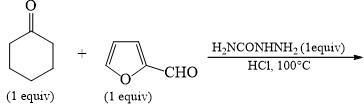Solution:
QUESTION: 4

The correct reagent for the following chemical conversions are: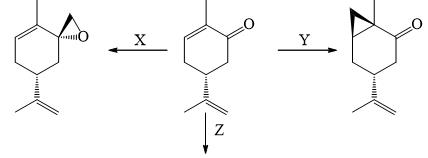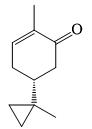Solution:
QUESTION: 5

In the following reaction, the major products [X] is: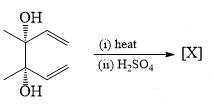Solution:
QUESTION: 6

The major product formed in the following reaction is: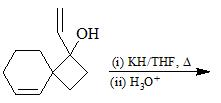Solution:
QUESTION: 7

The major products X and Y formed in the following reaction sequence are: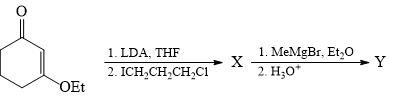Solution:
QUESTION: 8

Choose the correct reactivity order among following heterocyclic compounds (towards electrophilic substitution reaction)

I. Pyrrole; II. Furan; III. Thiopene; IV. Benzene

Solution:
QUESTION: 9

The major product formed in the following reaction is: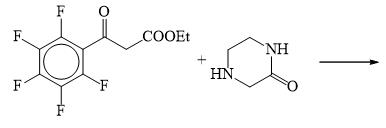Solution:
QUESTION: 10

Identify the major product of following reaction: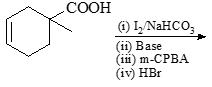Solution:
QUESTION: 11

The major product formed in the Beckmann rearrangement of the compound given below is: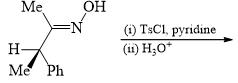Solution:
QUESTION: 12

The major product X and Y formed in the following reaction sequence are: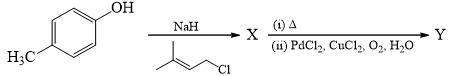Solution:
QUESTION: 13

The major product B formed in the following reaction sequence is: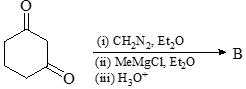Solution:
QUESTION: 14

The major product formed in the following reaction is: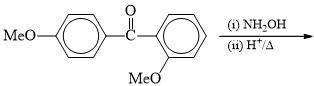Solution:
QUESTION: 15

In the following reaction sequence, the reagents X and Y are, respectively: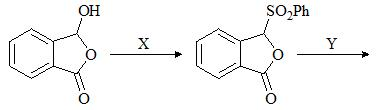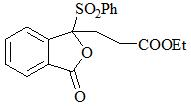Solution:
QUESTION: 16

Given that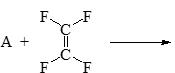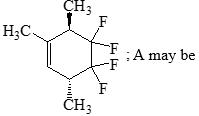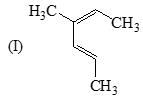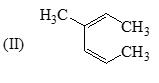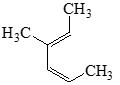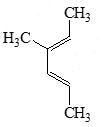Solution:
QUESTION: 17

The major product X and Y of the following reaction sequence are: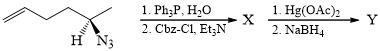Solution:
QUESTION: 18

For the following three step conversion of X to Y, the appropriate sequence of reactions is: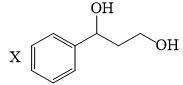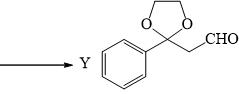Solution:
QUESTION: 19

Choose the correct product of the following reaction: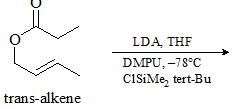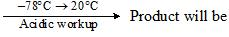Solution:
QUESTION: 20

The major product formed in the following reaction is:Solution:
QUESTION: 21

The product X and Y in the following reaction sequence are: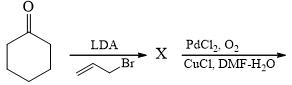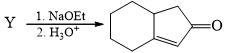Solution:
QUESTION: 22

Choose correct product in the following chemical reaction: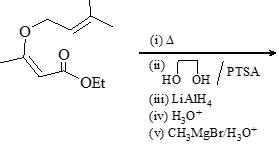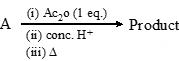Solution:
QUESTION: 23

The major product formed in the reaction of (S)-1, 2, 4-butanetriol with 3-pentanone in the presence of a catalytic amount of pTSA is:

Solution:
QUESTION: 24

Choose the correct product of the following reaction: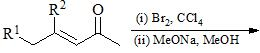Solution:
QUESTION: 25

Identify appropriate reagents X and Y in the following reactions: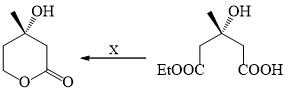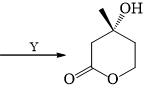Solution:
QUESTION: 26

The major product A & B of the following reactions are respectively: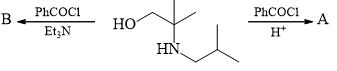Solution:
QUESTION: 27

Choose the correct product in the following reaction: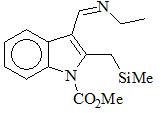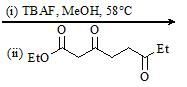Solution:
QUESTION: 28

Choose the correct product of the following reaction: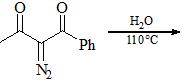Solution:
QUESTION: 29

In the reaction sequence, the structure of the major product Z and the overall yield for its formation from the ketone X, are: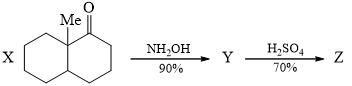Solution:
QUESTION: 30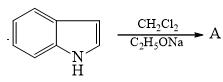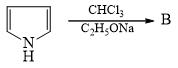Products A and B are

Solution:
*Multiple options can be correct
QUESTION: 31

Identify which compound give alkyne formation on reaction with PCl5 followed by alc. NaNH2 (excess):

Solution:
*Multiple options can be correct
QUESTION: 32

Correct reaction of the following is/are?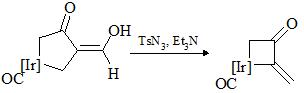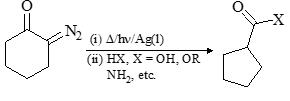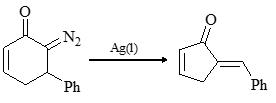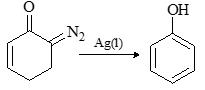Solution:
*Multiple options can be correct
QUESTION: 33

Choose the correct product(s):

Solution:
*Multiple options can be correct
QUESTION: 34

Choose the correct product(s) in the following chemical reaction:

Solution:
*Multiple options can be correct
QUESTION: 35

The following conversion reaction can be carried out by using reaction sequence/s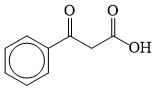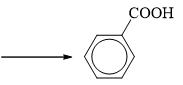Solution:
*Multiple options can be correct
QUESTION: 36

Which of the following reaction(s) give the same product?

Solution:
*Multiple options can be correct
QUESTION: 37

Methyl ketone may be prepared from:

Solution:
*Multiple options can be correct
QUESTION: 38

Which of the following reaction does not yield phenol?

Solution:
*Multiple options can be correct
QUESTION: 39

Which of the following statement(s) is/are correct about the following reaction: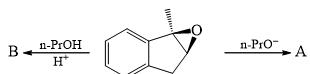Solution:
*Multiple options can be correct
QUESTION: 40

Which of the following compounds will give Hofmann elimination product?

Solution:
*Answer can only contain numeric values
QUESTION: 41

Number of correct chemical conversion(s) is/are: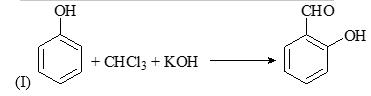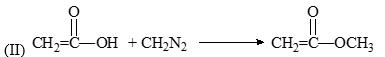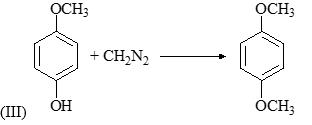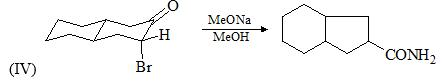Solution:
*Answer can only contain numeric values
QUESTION: 42

How many of the following chemical reactions represent correct product formation?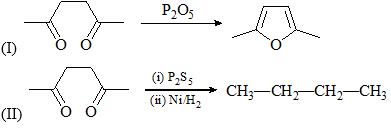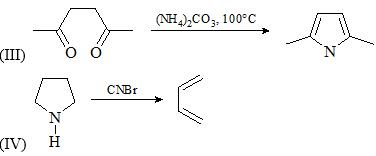Solution:
*Answer can only contain numeric values
QUESTION: 43

How many of the following chemical reactions can be used generate carbenes?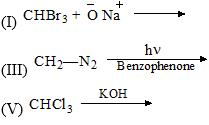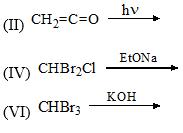Solution:
*Answer can only contain numeric values
QUESTION: 44

How many of the following chemical reaction represent correct product formation: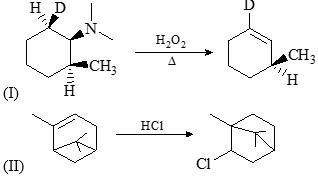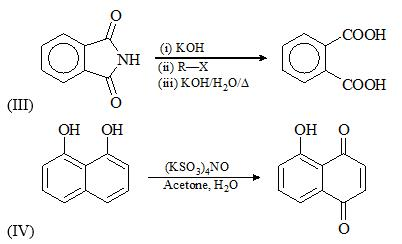Solution:
*Answer can only contain numeric values
QUESTION: 45

Predict correct number of chemical conversion(s):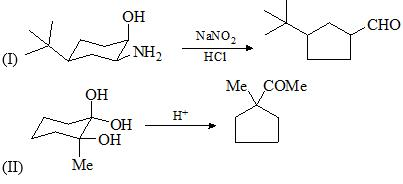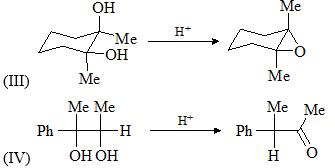Solution:
*Answer can only contain numeric values
QUESTION: 46

The number of carboxyl acid group(s) present in product R is/are: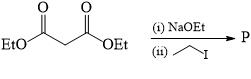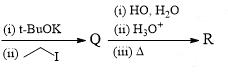Solution:
*Answer can only contain numeric values
QUESTION: 47

Among the following the number of reaction which shows carbene intermediate: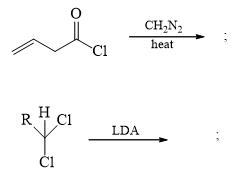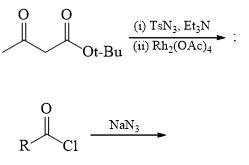Solution:
*Answer can only contain numeric values
QUESTION: 48

How many number of reaction proceed through NGP.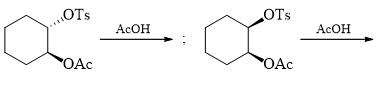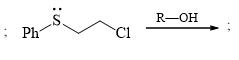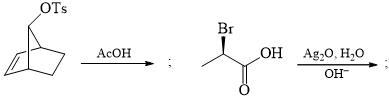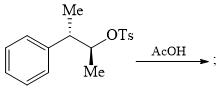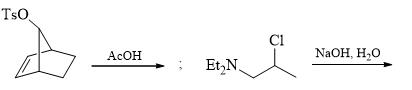Solution:
*Answer can only contain numeric values
QUESTION: 49

Number of reagents which correctly match in the reference to reaction on double bonds: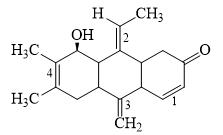i) CH2I2/ Zn-Cu                                              4

(ii) mCPBA (Peroxy acid)                              2

(iii) (PPh3)3RhCl/H2                                                    3

(iv) H2O2 / NaOH/H2O                                   1

(v) O3/Zn/H2O                                                4

(vi) Na/Liq.NH3/EtOH                                   2

(vii) CHCl3/KOH                                           3

(viii) PPh3/TMSI                                             1

Solution:
*Answer can only contain numeric values
QUESTION: 50

Number of correct statement about given reaction: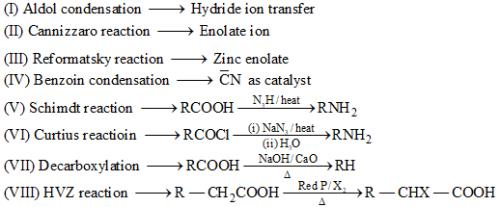Solution:
*Answer can only contain numeric values
QUESTION: 51

The total no. of compounds that form phenylhydrazone derivative under acidic conditions is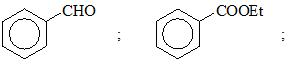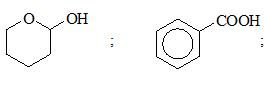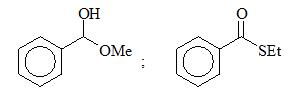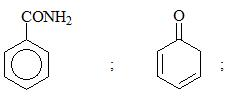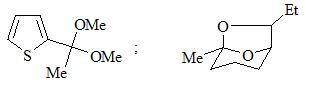Solution:
*Answer can only contain numeric values
QUESTION: 52

Which of the following compounds are aromatic compounds: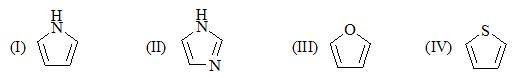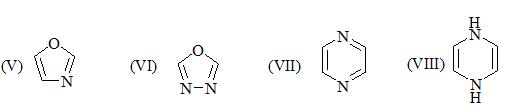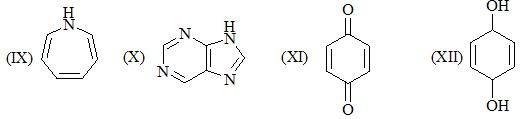Solution:
*Answer can only contain numeric values
QUESTION: 53

Amongst the following, the number of carboxylic acids which easily undergo decarboxylation on heating are: HCOOH, (COOH)2, CH2(COOH)2, C6H5COCH2COOH, C6H5COCOOH,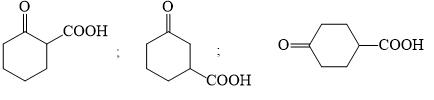Solution:
*Answer can only contain numeric values
QUESTION: 54

The total number of compounds that form a clear homogeneous solution on treatment with cold   dil. H2SO4 is _____________?

1-Propyne, Pyridne, Ethyl butanoate, THF, Propoxypropane, Propan-1-amine, Propane,             Cyclohexane, Cyclohexanone

Solution:
*Answer can only contain numeric values
QUESTION: 55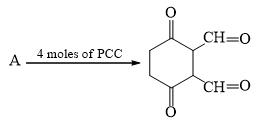Maximum number of moles of Ac2O consumed by reactant (A) is:

Solution:
*Answer can only contain numeric values
QUESTION: 56

Number of possible monoalkylated products formed in the Friedel-Craft reaction of anisole with 2-chloro-3-methylbutane in the presence of anhyd. AlCl3 at 50°C is _________?

Solution:
*Answer can only contain numeric values
QUESTION: 57

Z = Number of reaction in which Acetal is final product.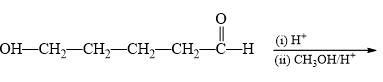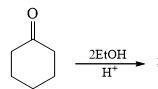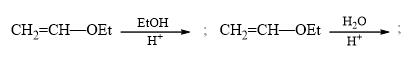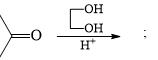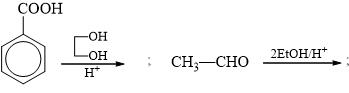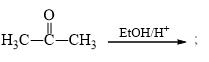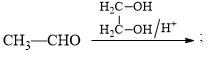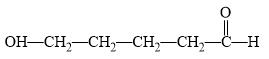Solution:
*Answer can only contain numeric values
QUESTION: 58

Among the following, the number of compounds, which can participate as ‘diene’ component in a Diels-Alder reaction is________: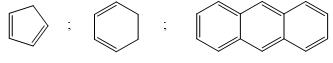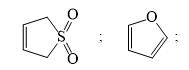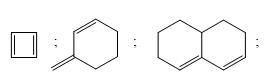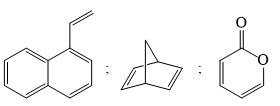Solution:
*Answer can only contain numeric values
QUESTION: 59

The compound isopentylnitrite is a source of NO+ ions and will react with an amine to generate a diazonium cation. Number of ‘oxygen atoms’ in the product formed is/are_____?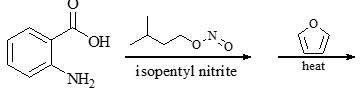Solution:
*Answer can only contain numeric values
QUESTION: 60

The total number of reactions in which condensation takes place: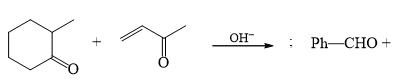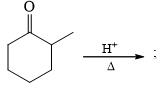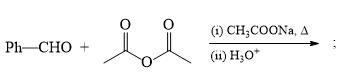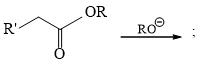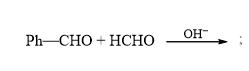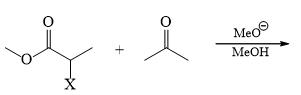Solution: